–Я–Њ–Є—Б–Ї –і–∞—В–∞—И–Є—В–∞ (1.687.043 –Ї–Њ–Љ–њ–Њ–љ–µ–љ—В–Њ–≤) –У–і–µ –Є—Б–Ї–∞—В—М–≤ –љ–∞–Є–Љ–µ–љ–Њ–≤–∞–љ–Є–Є–≤ –Њ–њ–Є—Б–∞–љ–Є–Є### Datasheet: 03P4MG (NEC)

300 Ma High-withstanding-voltage Mold Scr
–°–Ї–∞—З–∞—В—М:PDFZIP# Document Outline

• COVER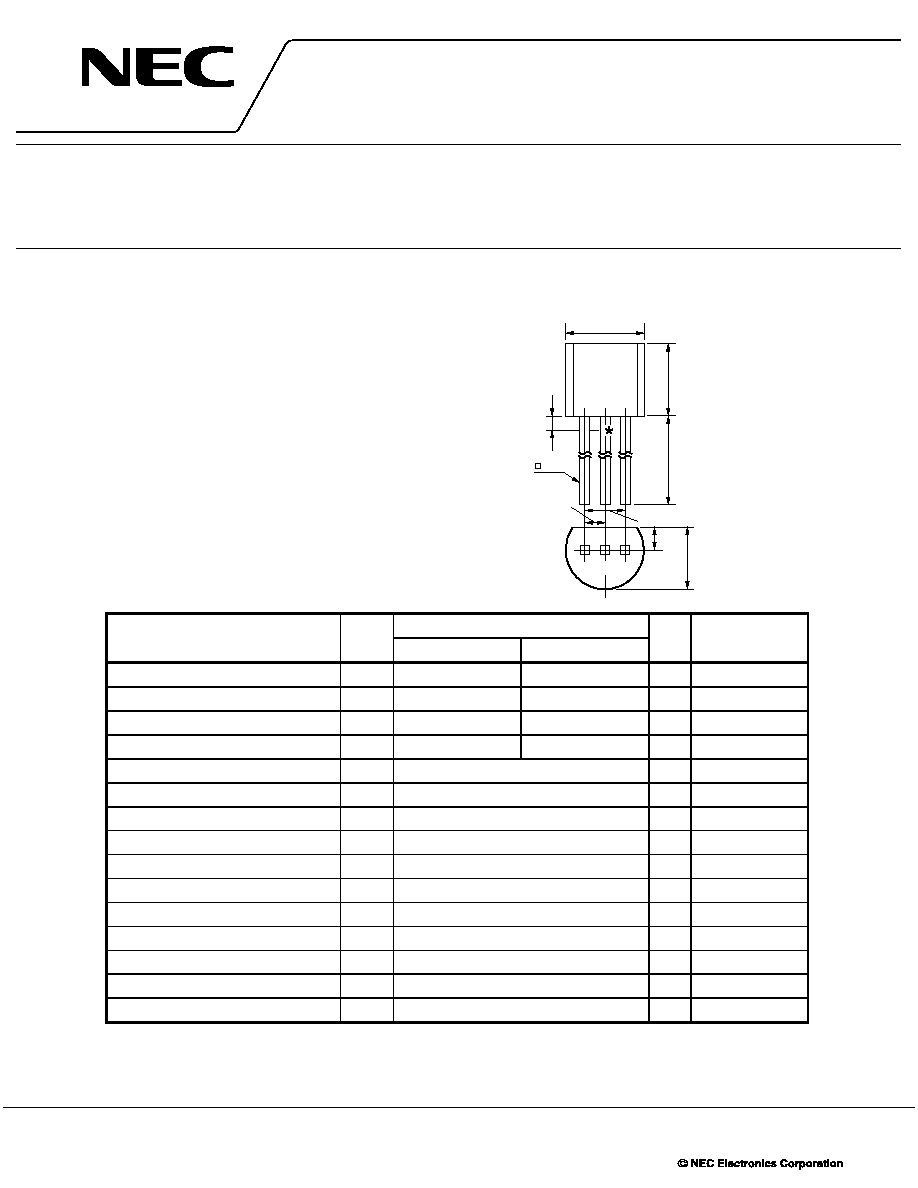The information in this document is subject to change without notice. Before using this document, please
sales representative for availability and additional information.
THYRISTORS
03P4MG,03P6MG
300 mA HIGH-WITHSTANDING-VOLTAGE MOLD SCR
DATA SHEET
Document No. D15290EJ4V0DS00 (4th edition)
Date Published February 2003 NS CP(K)
Printed in Japan
2002
The mark
5
5
5
5
shows major revised points.
DESCRIPTION
The 03P4MG and 03P6MG are P-gate fully diffused mold SCRs
with an average on-state current of 300 mA. The repeat peak
off-state voltages (and reverse voltages) are 400 and 600 V.
FEATURES
Ј
400 and 600 V high-withstanding-voltage series of products
Ј
The non-repetitive withstanding voltage is a high 700 V, making
it easy to harmonize the rise voltage of the surge absorber.
Ј
High-sensitivity thyristor (I
GT
= 3 to 50
µ
A)
Ј
Employs flame-retardant epoxy resin (UL94V-0)
APPLICATIONS
Leakage breakers, SSRs, various type of alarms, consumer
electronic equipments and automobile electronic components
ABSOLUTE MAXIMUM RATINGS (T
A
= 25∞C)
Parameter
Symbol
Ratings
Unit
Remarks
03P4MG
03P6MG
Non-repetitive Peak Reverse Voltage
V
RSM
700
700
V
R
GK
= 1 k
Non-repetitive Peak Off-state Voltage
V
DSM
700
700
V
R
GK
= 1 k
Repetitive Peak Reverse Voltage
V
RRM
400
600
V
R
GK
= 1 k
Repetitive Peak Off-state Voltage
V
DRM
400
600
V
R
GK
= 1 k
Average On-state Current
I
T(AV)
300 (T
A
= 30∞C, Single half-wave,
= 180∞)
mA
Refer to Figure 10.
Effective On-state Current
I
T(RMS)
470
mA
-
Surge On-state Current
I
TSM
8 (f = 50 Hz, Sine half-wave, 1 cycle)
A
Refer to Figure 2.
Fusing Current
i
T
2
dt
0.15 (1 ms
t
10 ms)
A
2
s
-
Critical Rate of On-state Current of Rise
dI
T
/dt
20
A/
µ
s
-
Peak Gate Power Dissipation
P
GM
100 (f
50 Hz, Duty
10%)
mW
Refer to Figure 3.
Average Gate Power Dissipation
P
G(AV)
10
mW
Refer to Figure 3.
Peak Gate Forward Current
I
FGM
100 (f
50 Hz, Duty
10%)
mA
-
Peak Gate Reverse Voltage
V
RGM
6
V
-
Junction Temperature
T
j
-
40 to +125
∞C
-
Storage Temperature
T
stg
-
55 to +150
∞C
-
PACKAGE DRAWING (Unit: mm)
1.27
2.54
1.77 MAX.
4.2 MAX.
1
3
1.5
12.7 MIN.
5.5 MAX.
5.2 MAX.
0.5
2
Electrode connection
1: Gate
2: Anode
3: Cathode
*T
C
test bench-mark
Standard weight: 0.3 g
5
5
5
5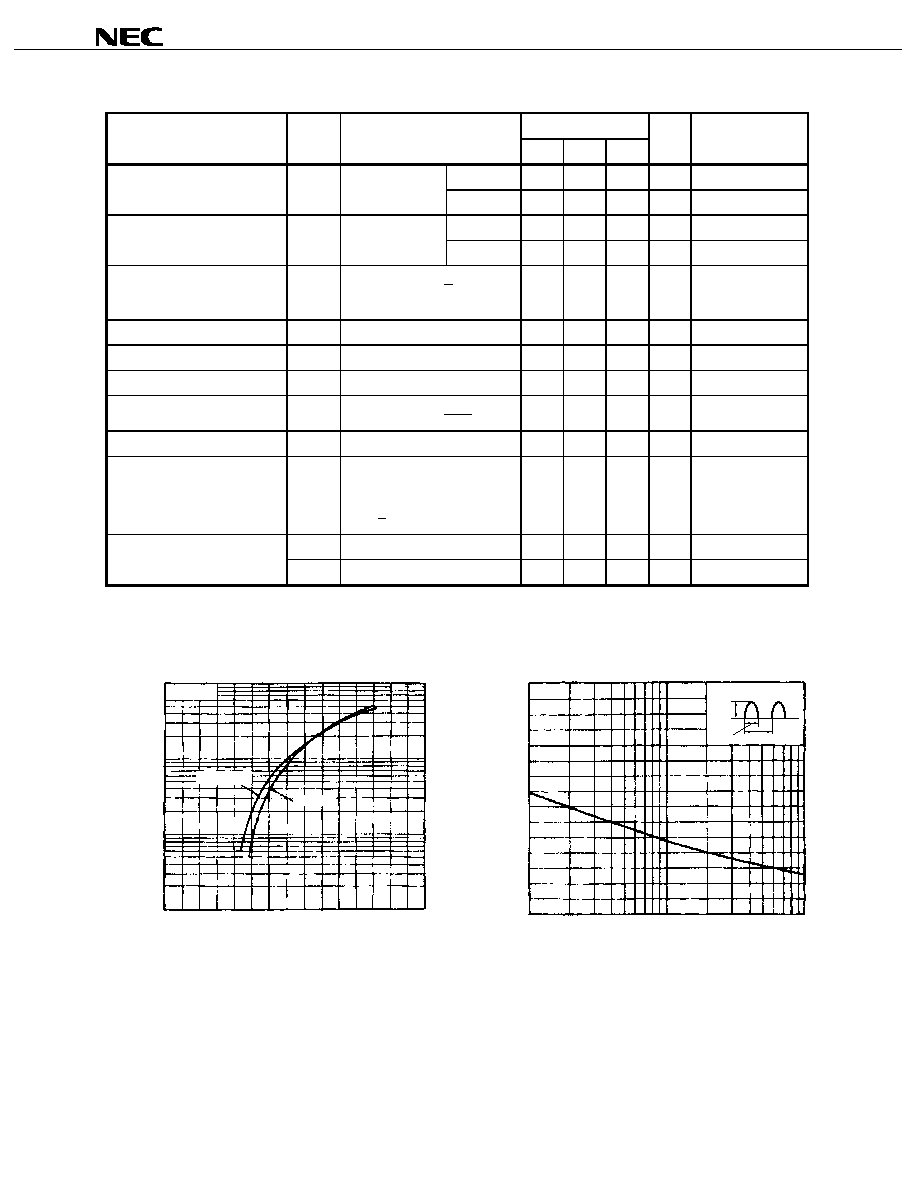Data Sheet D15290EJ4V0DS
2

03P4MG,03P6MG
ELECTRICAL CHARACTERISTICS (T
j
= 25∞C, R
GK
= 1 k
)
Parameter
Symbol
Conditions
Specifications
Unit
Remarks
MIN.
TYP.
MAX.
Non-repetitive Peak Reverse
I
RRM
V
RM
= V
RRM
T
j
= 25∞C
-
-
10
µ
A
-
Current
T
j
= 125∞C
-
-
100
µ
A
-
Non-repetitive Peak Off-state
I
DRM
V
DM
= V
DRM
T
j
= 25∞C
-
-
10
µ
A
-
Current
T
j
= 125∞C
-
-
100
µ
A
-
Critical Rate-of-rise of Off-state
dV
D
/dt
10
-
-
V/
µ
s
-
Voltage
T
j
= 125∞C, V
DM
=
3
2
V
DRM
On-state Voltage
V
T
I
T
= 4 A
-
-
2.2
V
Refer to Figure 1.
Gate Trigger Current
I
GT
V
DM
= 6 V, R
L
= 100
3
-
50
µ
A
-
Gate Trigger Voltage
V
GT
V
DM
= 6 V, R
L
= 100
-
-
0.8
V
-
Gate Non-trigger Voltage
V
GD
T
j
= 125∞C, V
DM
=
2
V
DRM
0.2
-
-
V
-
Holding Current
I
H
V
DM
= 24 V, I
TM
= 4 A
-
-
5
mA
-
Turn-off Time
t
q
T
j
= 125∞C, I
T
= 200 mA,
-
60
-
µ
s
-
dI
R
/dt = 15 A/
µ
s, V
R
25 V,
V
DM
=
2
3 V
DRM
, dV
D
/dt = 10 V/
µ
s
Thermal Resistance
R
th(j-C)
Junction-to-case DC
-
-
50
∞C/W
Refer to Figure 14.
R
th(j-A)
Junction-to-ambient DC
-
-
230
∞C/W
Refer to Figure 14.
TYPICAL CHARACTERISTICS (T
A
= 25∞C)
Figure 1. i
T
vs.

T
Characteristics
Figure 2. I
TSM
Rating
O
n
-
s
ta
te
C
u
r
r
e
n
t
i
T
(m
A
)
MAX.
0
1
2
3
T
j
= 125∞C
25∞C
10000
1000
100
10
On-state Voltage
T
(V)
S
u
rge On-st
a
t
e
Current
I
TS
M
(A
)
At initial, T
j
= 125∞C
I
TSM
10 ms 20 ms
14
12
10
8
6
4
2
0
1
10
100
5
50
Cycles (N)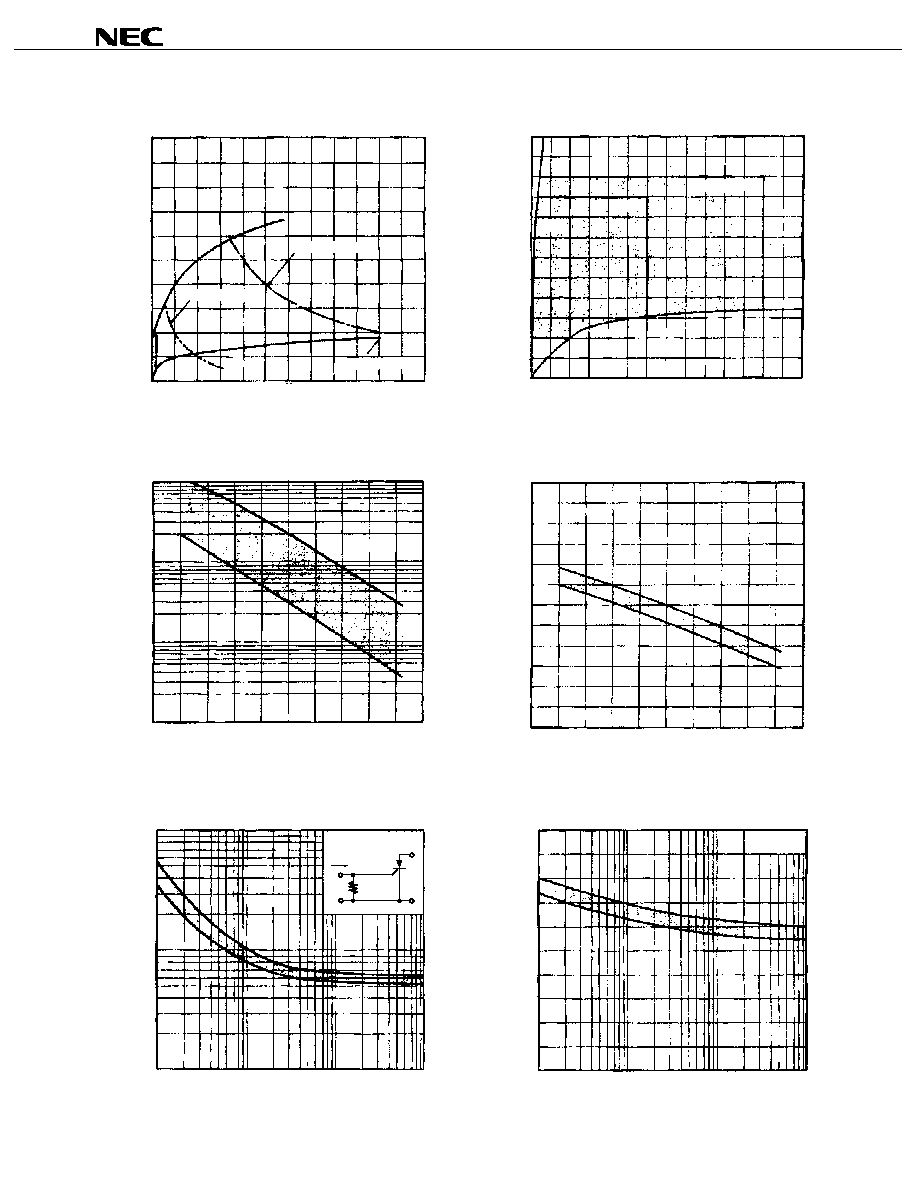Data Sheet D15290EJ4V0DS
3

03P4MG,03P6MG
Figure 3. Gate Rating
Figure 4. Example of Gate Characteristics
Gat
e
Forw
ard V
o
l
t
age
V
FG
(V
)
P
G(AV)
= 10 mW
P
GM
= 100 mW
I
FG
= 100 mA
5.0
4.0
3.0
2.0
1.0
0
0
20
40
60
80
100
120
Gate Forward Current I
FG
(mA)
Gat
e
Tri
gger
V
o
l
t
age
V
GT
(V
)
T
j
=
-
40∞C
0∞C
25∞C
1.2
1.0
0.8
0.6
0.4
0.2
0
0
100
200
300
50
150
250
350
Gate Trigger Current I
GT
(
µ
A)
Figure 5. I
GT
vs. T
A
Example of Characteristics
Figure 6. V
GT
vs. T
A
Example of Characteristics
Gat
e
Tri
gger Current

I
GT
(
µ
A)
100
10
1
0.1
-
40
-
20
0
40
80
20
60
100
140
120
Ambient Temperature T
A
(∞C)
Gat
e
Tri
gger
V
o
l
t
age
V
GT
(V
)
-
40
-
20
0
40
80
20
60
100
140
120
1.2
1.0
0.8
0.6
0.2
0.4
0
Ambient Temperature T
A
(∞C)
Figure 7. i
GS
vs.

Example of Characteristics
Figure 8.

GT
vs.

Example of Characteristics
S
uppl
y Gat
e
Tri
gger Current
i
GS
(m
A
)
T
A
= 25∞C
i
GS
R
GK
1 k
10
5
1
0.5
0.1
1
10
1000
100
Pulse Width
(
µ
s)
Gat
e
Tri
gger
V
o
l
t
age
GT
(V
)
T
A
= 25∞C
1
10
1000
100
1.0
0.8
0.6
0.4
0.2
0
Pulse Width
(
µ
s)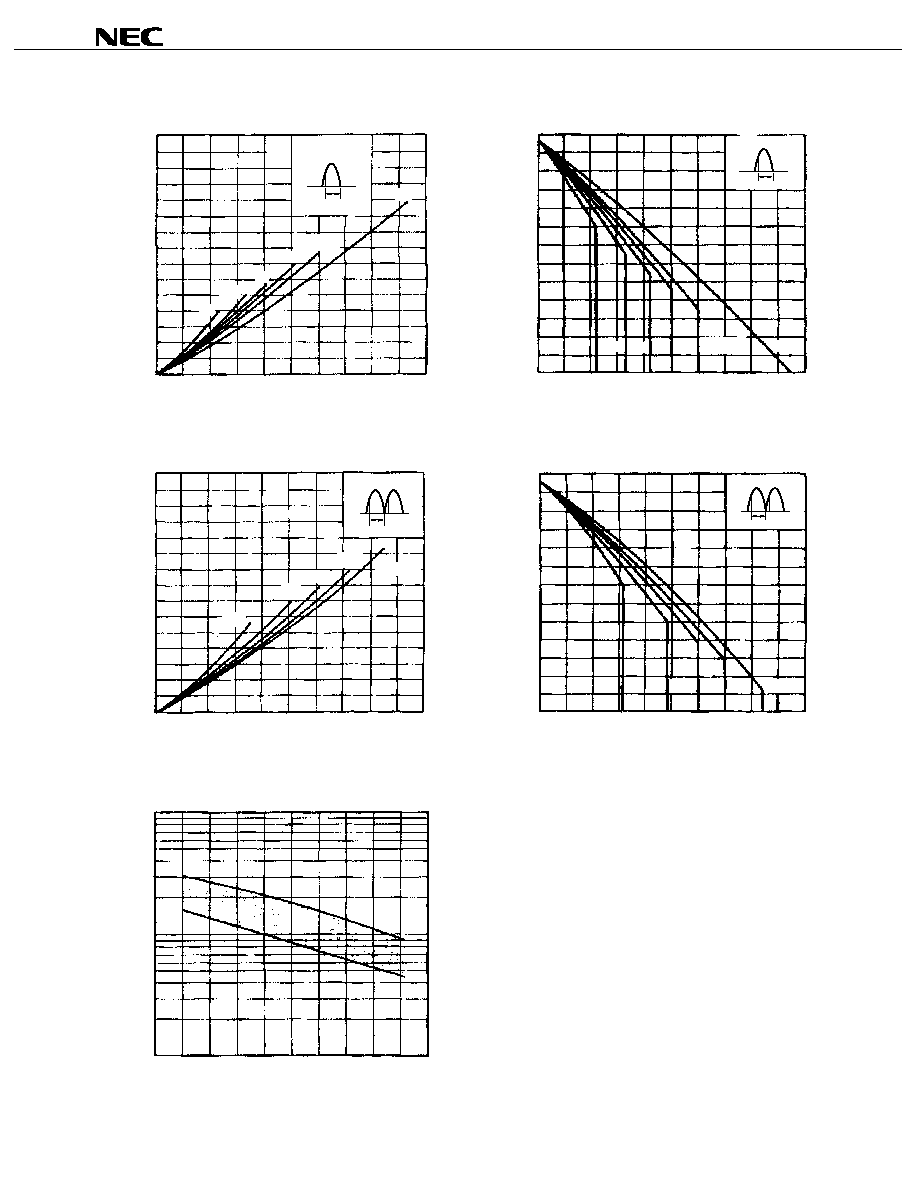Data Sheet D15290EJ4V0DS
4

03P4MG,03P6MG
Figure 9. P
T(AV)
vs. I
T(AV)
Characteristics
Figure 10. T
A
vs. I
T(AV)
Characteristics
A
v
erage On-s
t
a
t
e
P
o
w
e
r Di
ss
i
pat
i
o
n
P
T(
A
V
)
(W
)
30∞
60∞
90∞
120∞
DC
Single Half-wave
0.7
0.6
0.5
0.4
0.3
0.2
0.1
0
0
100
200
400
300
500
= 180∞
Average On-state Current I
T(AV)
(mA)
A
m
bi
ent
Te
m
pera
t
ure T
A
(∞
C)
30∞
60∞ 90∞ 120∞
DC
Single Half-wave
120
100
80
60
40
20
0
0
100
200
400
300
500
= 180∞
Average On-state Current I
T(AV)
(mA)
Figure 11. P
T(AV)
vs. I
T(AV)
Characteristics
Figure 12. T
A
vs. I
T(AV)
Characteristics
A
v
erage On-s
t
a
t
e
P
o
w
e
r Di
ss
i
pat
i
o
n
P
T(
A
V
)
(W
)
Single Full-wave
0.7
0.6
0.5
0.4
0.3
0.2
0.1
0
0
100
200
400
300
500
= 180∞
30∞
60∞
90∞
120∞
Average On-state Current I
T(AV)
(mA)
A
m
bi
ent
Te
m
pera
t
ure T
A
(∞
C)
Single Full-wave
30∞
60∞
90∞ 120∞
120
100
80
60
40
20
0
0
100
200
400
300
500
= 180∞
Average On-state Current I
T(AV)
(mA)
Figure 13. I
H
vs. T
A
Example of Characteristics
Hol
d
i
ng Current
I
H
(m
A
)
10
5
1
0.5
0.1
-
40
-
20
0
40
80
20
60
100
140
120
Ambient Temperature T
A
(∞C)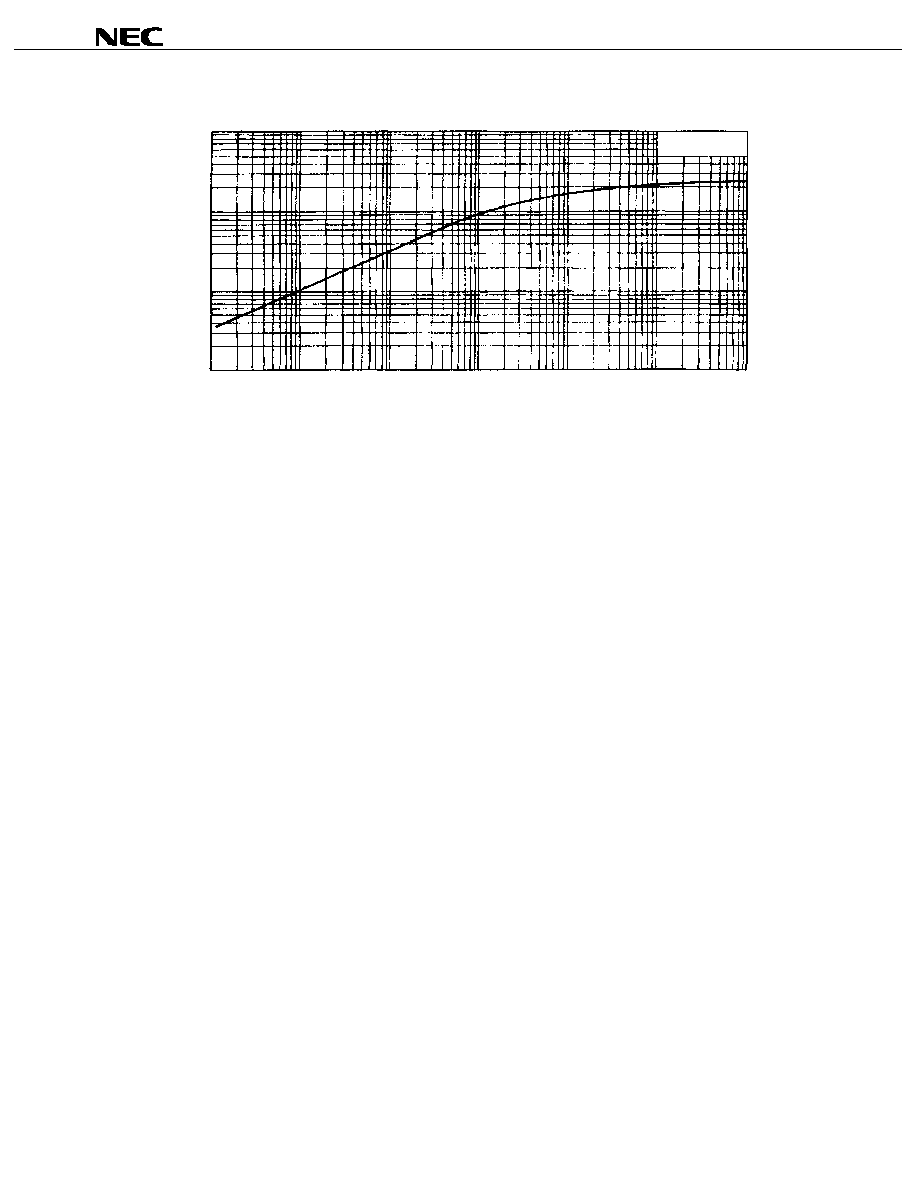Data Sheet D15290EJ4V0DS
5

03P4MG,03P6MG
Figure 14. Z
th
Characteristics
Trans
i
ent
Ther
m
a
l
I
m
pedan
c
e
Z
th
(∞
C
/
W
)
1000
100
10
1
0.001
0.01
0.1
1
10
100
1000
Connection to
ambient
Time t (s)